﻿ Principle of Numerical Solution of Consolidation | Consolidation | GEO5 | Online Βοήθεια

To link στάλθηκε στο email σας.

Ήταν αδύνατο να στείλουμε το link στο email σας. Παρακαλώ ελέγξτε το email σας.

# Online Βοήθεια

Tree
Settings
Προϊόντα:
προγράμματα:
Γλώσσα:

## Consolidation

In standard stress analysis the program GEO5 FEM allows for two specific approaches to the modeling of pore pressure action on a soil body. In case of undrained conditions it is assumed that all boundaries surrounding the undrained soil are impermeable, the soil is considered as volumetrically incompressible, and the applied load results in the generation of excess pore pressure within this layer. Introducing suitable boundary conditions that allow for a gradual dissipation of excess pore pressure provides passage to drained conditions. In case of drained conditions we assume that the resulting pore pressure is no longer influenced by the deformation of the soil body. Transition from drained to undrained conditions describes the theory of consolidation.

The term consolidation stands for the soil deformation in time caused by external load, which can be either constant or time dependent. This is a reological process. For the present case we limit our attention to so called primary consolidation characterized by the reduction of volume of pores and thus the change of the internal soil structure due to load accompanied by the escape of water from pores. The analysis assumes a fully saturated soil. Consolidation analysis in a partially saturated soil is not addressed by the program. The governing equation describing the flow of water (continuity equation,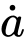represents a time derivative of a given quantity) in a fully saturated (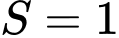,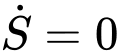) deforming soil body is provided by (recall the Richards equation for the description of transient water flow).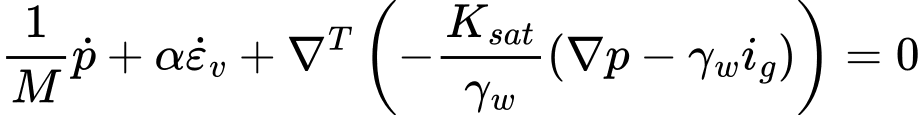where: M - Biot‘s modulus, assumed in the range of M = (100-1000)Ksk (Ksk is unit modulus of skeleton). In general, it is a large number enforcing a volumetric incompressibility of a given fully saturated soil at very short times at the onset of consolidation. A default setting is M = 106 kPa. α - Biot’s parameter, typically assumed α = 1 p - pore pressure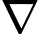p - pore pressure gradient Ksat - permeability matrix storing coefficients of permeability for a fully saturated soil, typical values for selected soils are stored in Table ig - hydraulic gradient

The rate of change of total pressure is given by: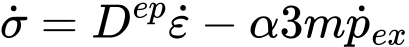where: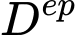- current stiffness matrix pex - excess pore pressure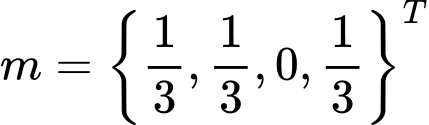- for plane strain or axial symmetry

Note that the total pore pressure p is a sum of steady state pore pressure pss and excess pore pressure pex. It holds: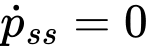The continuity equation (1) can be thus written as: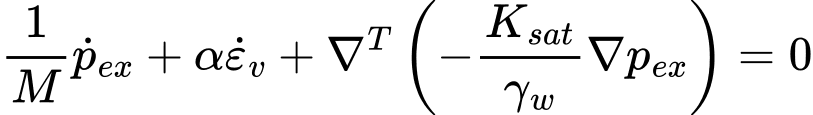adopting the zero excess pore pressure boundary condition at the boundary with prescribed pressure as: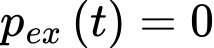and the zero flow (q(t) = 0) across the boundary with prescribed water flux density:where: n - components of outward unit normal

See: Setting hydraulic boundary conditions.

The overall total stress is then provided by: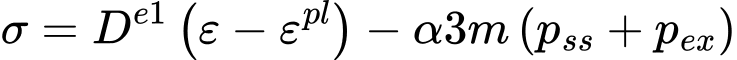where: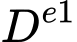- elastic stiffness matrix ε - vector of overall strains εpl - vector of overall plastic strains

The current values of strains and excess pore pressure in equation (7) follow from the application of static equations of equilibrium and continuity equation (4) within the solution of coupled stress and water transport problem using the principal of virtual displacements. As in case of transient water flow analysis a fully implicit forward Euler method is adopted to perform time discretization of equation (4). Further details can be found in [1,2,3].

## Consolidation analysis

As in case of transient water flow analysis the first calculation stage serves to set initial conditions, i.e. the distribution of geostatic stress and steady state pore pressure. The steady state pressure values also equal to the overall pressure values at the end of consolidation. The initial pore pressure values are set by specifying the groundwater table (GWT) only. It is worth to note that even if GWT table is found inside the analyzed soil body the soil below and above GWT is assumed fully saturated. This applies also to soils being introduced into the analysis in subsequent calculation stages (activating new regions). Removing or excavating soils (deactivating existing regions) is not possible with the current version of the program. The actual consolidation analysis is performed from the second stage on and requires setting the hydraulic boundary conditions, setting the time duration of a given calculation stage, setting the expected number of time steps and setting the way of introducing the load in to the analysis.

## Setting hydraulic boundary conditions

The program allows for introducing two types of hydraulic boundary conditions, recall equations (5) and (6):

• Zero pore pressure condition (p = 0), which allows for free water outflow from the soil body, i.e. condition representing a permeable boundary. More specifically, this condition corresponds to the zero value of the excess pore pressure pex. The overall value of pore pressure along this boundary is thus equal to pss. This is a default boundary condition and is it assumed along all external domain boundaries, therefore also along the external boundaries of new regions.
• Zero flux density condition (no inflow/outflow, q = 0), i.e. condition representing an impermeable boundary. If needed, this condition has to be introduced manually.

The choice of a given boundary condition influences the rate of consolidation. Further details can be found in .

## Setting the time step size - expected number of time steps in a given stage

Unlike the transient water flow analyses the initial time step size (discrete value of time increment when solving equation (4)) is in case of consolidation not directly assigned. Instead, this step is set on the bases of the specified duration of calculation stage and the input number of time steps expected for a given stage. In case of linear consolidation (all soils are assumed linearly elastic) the input number of time steps is performed. A time step reduction can take place in case of nonlinear consolidation if the lack of convergence for the current time step is encountered. This increases the number of steps to complete the stage analysis. When specifying the number of steps in relation to the stage duration one should keep in mind that at the onset of consolidation the time step should be relatively small (particularly when referring to a load stage in conjunction with nonlinear consolidation), while with gradual progress of consolidation the time step size may reach several tens of days. Further details can be found in .

## Introducing load into the analysis

As in case of transient flow analysis the program offers two options only:

• The load is applied at one step at the beginning of the calculation stage. More specifically, a linear increase of load over the first time step is assumed. Thus if we are interested in the behavior at t → 0, it is necessary to suitably choose the combination of number of time steps and duration of the first stage (e.g. 1 and 0.001) . In case of a very short time step and impermeable boundaries (q = 0) we simulate the response of volumetrically incompressible soil (K → ∞) with a finite value of the shear modulus. The results for t → 0 will then agree well with the results derived from standard stress analysis with undrained soils. Further details can be found in .
• The load linearly increases over the calculation stage. The load increment then depends on the current time step size. Especially in case of nonlinear consolidation one should properly specify the time span over which the load is introduced to avoid convergence difficulties.

Providing there is no load change within a given stage the above setting options are irrelevant.

## Application of beam elements in consolidation

The beam permeability depends on its location and the choice of hydraulic boundary conditions. A beam found inside the soil body is in its normal direction impermeable. On domain boundaries the beam permeability in normal direction, as in case of water flow analyses, is driven by the selected boundary condition. In case of permeable boundary (p = 0) the beam on this boundary is fully permeable, while in case of impermeable boundary (q = 0) the beam on this boundary is also impermeable.

## Application of contact elements in consolidation

The reason for introducing contact elements into the analysis is twofold. First, we wish to allow for a relative mutual shift between two soils, soil and rock or soil and beam element, e.g. in the analysis of interaction of soil and sheeting structure. Second, the aim is at modeling potential drain along the beam or in general along a line to which the contact element is assigned. In every case one should realize a coupled simulation of both phenomena, i.e. stress and water flow analysis being carried out simultaneously. If not specified otherwise the program assumes the flow within a contact element being dependent on permeabilities of surrounding soils both in the longitudinal and transverse direction. In case of contact attached to the beam element the normal permeability kn is irrelevant as the beam is assumed in this direction either fully impermeable (kn = 0) or fully permeable (kn → ∞), see "Application of beam elements in consolidation".

Time evolution of individual variables, e.g. settlement or excess pore pressure, will be in case of linear consolidation always bounded by the solution of stress analysis when considering either undrained soils (all active soils in the analyzed domain are specified as undrained) or drained soils (standard setting, all active soils in the analyzed domain are specified as drained). The latter case coincides with the steady state analysis with total dissipation of excess pore pressure. The results of linear stress analysis with drained soils and linear consolidation derived at t → ∞ must be identical. However, this does not hold for nonlinear analyses as in such cases we cannot rely on the principle of superposition. Further details can be found in .

Unlike water flow analyses, the solution of consolidation requires application of higher order elements (e.g. 6-node triangular or 8-node quadrilateral elements). While displacements are calculated at all nodes of a given element (quadratic approximation of displacement field), pore pressure is calculated at the corner nodes only (linear approximation of pore pressure).

Unlike one-dimensional consolidation implemented in program "Settlement" the two-dimensional consolidation yields at t → 0 zero volumetric strain and thus also zero mean effective stress only. Individual components of the displacement field are generally nonzero.

Literature:

 M. Šejnoha, T. Janda, H. Pruška, M. Brouček, Metoda konečných prvků v geomechanice: Teoretické základy a inženýrské aplikace, předpokládaný rok vydání (2015)

 Z. Bittnar and J. Šejnoha, Numerické Metody Mechaniky II. České vysoké učení technické v Praze, 1992

 Z. Bittnar and J. Šejnoha, Numerical methods in structural engineering, ASCE Press, 1996